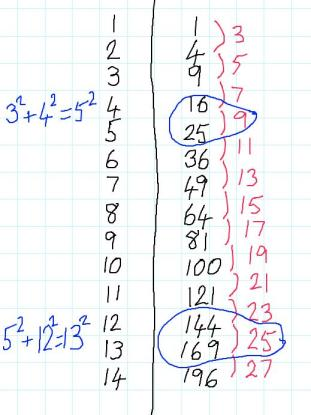# Generating TriplesIf the sides of a right angled triangle are $a$, $b$ and $c$ units, with $c$ being the hypotenuse or longest side, we know by Pythagoras' Theorem that $a^2+b^2=c^2$.

Charlie has been looking for examples where $a$, $b$ and $c$ are all whole numbers. These sets are called Pythagorean Triples.

He drew a table:From his table, he spotted two sets of Pythagorean triples:

$3^2+4^2=5^2$

$5^2+12^2=13^2$

Can you find more Pythagorean Triples like Charlie's?

Can you find a formula to generate them?
Can you prove that your formula works?

Alison has been working on Pythagorean Triples where the hypotenuse is 2 units longer than one of the other sides.
So far, she has found these:
$4^2 + 3^2 = 5^2$

$6^2+8^2=10^2$

$8^2+15^2=17^2$

Some of these are just scaled-up versions of Charlie's triples, but some of them are new and can't be divided by a common factor (these are called primitive triples).

Can you find more Pythagorean Triples like Alison's?

Can you find a formula for generating Pythagorean Triples like Alison's?
Can you prove that your formula works?

Here are some other questions you might like to consider:

• Can you find triples where the hypotenuse is 3 units longer than one of the other sides? Or 4 units longer? Or...?
• Can you say anything about when such triples will be primitive triples?
• Are there any triples where the two shorter sides differ by one unit?

I noticed that if $a^2 + b^2 = (b+2)^2$, a and b have nothing in common but the hypotenuse is always two more than one of the other sides.